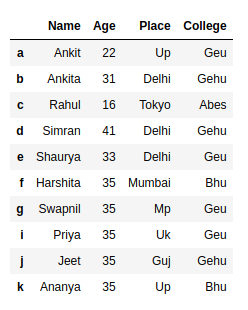Related Articles
Count all rows or those that satisfy some condition in Pandas dataframe
• Last Updated : 10 Jul, 2020

Let’s see how to count number of all rows in a Dataframe or rows that satisfy a condition in Pandas.

1) Count all rows in a Pandas Dataframe using Dataframe.shape.

`Dataframe.shape` returns tuple of shape (Rows, columns) of dataframe/series.

Let’s create a pandas dataframe.

 `# import pandas library as pd``import` `pandas as pd`` ` `# List of Tuples``students ``=` `[(``'Ankit'``, ``22``, ``'Up'``, ``'Geu'``),``           ``(``'Ankita'``, ``31``, ``'Delhi'``, ``'Gehu'``),``           ``(``'Rahul'``, ``16``, ``'Tokyo'``, ``'Abes'``),``           ``(``'Simran'``, ``41``, ``'Delhi'``, ``'Gehu'``),``           ``(``'Shaurya'``, ``33``, ``'Delhi'``, ``'Geu'``),``           ``(``'Harshita'``, ``35``, ``'Mumbai'``, ``'Bhu'` `),``           ``(``'Swapnil'``, ``35``, ``'Mp'``, ``'Geu'``),``           ``(``'Priya'``, ``35``, ``'Uk'``, ``'Geu'``),``           ``(``'Jeet'``, ``35``, ``'Guj'``, ``'Gehu'``),``           ``(``'Ananya'``, ``35``, ``'Up'``, ``'Bhu'``)``            ``]`` ` `# Create a DataFrame object from``# list of tuples with columns``# and indices.``details ``=` `pd.DataFrame(students, columns ``=``[``'Name'``, ``'Age'``, ``                                           ``'Place'``, ``'College'``],``                        ``index ``=``[``'a'``, ``'b'``, ``'c'``, ``'d'``, ``'e'``,``                                ``'f'``, ``'g'``, ``'i'``, ``'j'``, ``'k'``])`` ` `details`

Output:Code: Count all rows

 `# import pandas library as pd``import` `pandas as pd`` ` `# List of Tuples``students ``=` `[(``'Ankit'``, ``22``, ``'Up'``, ``'Geu'``),``           ``(``'Ankita'``, ``31``, ``'Delhi'``, ``'Gehu'``),``           ``(``'Rahul'``, ``16``, ``'Tokyo'``, ``'Abes'``),``           ``(``'Simran'``, ``41``, ``'Delhi'``, ``'Gehu'``),``           ``(``'Shaurya'``, ``33``, ``'Delhi'``, ``'Geu'``),``           ``(``'Harshita'``, ``35``, ``'Mumbai'``, ``'Bhu'` `),``           ``(``'Swapnil'``, ``35``, ``'Mp'``, ``'Geu'``),``           ``(``'Priya'``, ``35``, ``'Uk'``, ``'Geu'``),``           ``(``'Jeet'``, ``35``, ``'Guj'``, ``'Gehu'``),``           ``(``'Ananya'``, ``35``, ``'Up'``, ``'Bhu'``)``            ``]`` ` `# Create a DataFrame object from``# list of tuples with columns``# and indices.``details ``=` `pd.DataFrame(students, columns ``=``[``'Name'``, ``'Age'``,``                                           ``'Place'``, ``'College'``],``                        ``index ``=``[``'a'``, ``'b'``, ``'c'``, ``'d'``, ``'e'``, ``                                ``'f'``, ``'g'``, ``'i'``, ``'j'``, ``'k'``])`` ` `# 0th index of tuple returned by shape``# attribute give the number``# of rows in a given dataframe``num_rows ``=` `details.shape[``0``]`` ` `print``(``'Number of Rows in given dataframe : '``,``      ``num_rows)`

Output:

`Number of Rows in given dataframe :  10`

2) Count all rows in a Pandas Dataframe using Dataframe.index.

`Dataframe.index` attribute gives a sequence of index or row labels.

Code:

 `import` `pandas as pd`` ` `# List of Tuples``students ``=` `[(``'Ankit'``, ``22``, ``'Up'``, ``'Geu'``),``           ``(``'Ankita'``, ``31``, ``'Delhi'``, ``'Gehu'``),``           ``(``'Rahul'``, ``16``, ``'Tokyo'``, ``'Abes'``),``           ``(``'Simran'``, ``41``, ``'Delhi'``, ``'Gehu'``),``           ``(``'Shaurya'``, ``33``, ``'Delhi'``, ``'Geu'``),``           ``(``'Harshita'``, ``35``, ``'Mumbai'``, ``'Bhu'` `),``           ``(``'Swapnil'``, ``35``, ``'Mp'``, ``'Geu'``),``           ``(``'Priya'``, ``35``, ``'Uk'``, ``'Geu'``),``           ``(``'Jeet'``, ``35``, ``'Guj'``, ``'Gehu'``),``           ``(``'Ananya'``, ``35``, ``'Up'``, ``'Bhu'``)``            ``]`` ` `# Create a DataFrame object from``# list of tuples with columns``# and indices.``details ``=` `pd.DataFrame(students, columns ``=``[``'Name'``, ``'Age'``,``                                           ``'Place'``, ``'College'``],``                        ``index ``=``[``'a'``, ``'b'``, ``'c'``, ``'d'``, ``'e'``,``                                ``'f'``, ``'g'``, ``'i'``, ``'j'``, ``'k'``])`` ` `# count number of rows in given dataframe ``# by finding the length of indices``num_rows ``=` `len``(details.index)`` ` `print``(``'Number of Rows in given dataframe : '``,``      ``num_rows)`

Output:

`Number of Rows in given dataframe :  10`

3) Count rows in a Pandas Dataframe that satisfies a condition using Dataframe.apply().

`Dataframe.apply()`, apply function to all the rows of a dataframe to find out if elements of rows satisfies a condition or not, Based on the result it returns a bool series.

Code:

 `# import pandas library as pd``import` `pandas as pd`` ` `# List of Tuples``students ``=` `[(``'Ankit'``, ``22``, ``'Up'``, ``'Geu'``),``           ``(``'Ankita'``, ``31``, ``'Delhi'``, ``'Gehu'``),``           ``(``'Rahul'``, ``16``, ``'Tokyo'``, ``'Abes'``),``           ``(``'Simran'``, ``41``, ``'Delhi'``, ``'Gehu'``),``           ``(``'Shaurya'``, ``33``, ``'Delhi'``, ``'Geu'``),``           ``(``'Harshita'``, ``35``, ``'Mumbai'``, ``'Bhu'` `),``           ``(``'Swapnil'``, ``35``, ``'Mp'``, ``'Geu'``),``           ``(``'Priya'``, ``35``, ``'Uk'``, ``'Geu'``),``           ``(``'Jeet'``, ``35``, ``'Guj'``, ``'Gehu'``),``           ``(``'Ananya'``, ``35``, ``'Up'``, ``'Bhu'``)``            ``]`` ` `# Create a DataFrame object from``# list of tuples with columns``# and indices.``details ``=` `pd.DataFrame(students, columns ``=``[``'Name'``, ``'Age'``,``                                           ``'Place'``, ``'College'``],``                        ``index ``=``[``'a'``, ``'b'``, ``'c'``, ``'d'``, ``'e'``, ``                                ``'f'``, ``'g'``, ``'i'``, ``'j'``, ``'k'``])`` ` `# Get a bool series representing which row``# satisfies the condition i.e. True for``# row in which 'College' is 'Geu'``details ``=` `details.``apply``(``lambda` `x : ``True``            ``if` `x[``'College'``] ``=``=` `"Geu"` `else` `False``, axis ``=` `1``)`` ` `# Count number of True in the series``num_rows ``=` `len``(details[details ``=``=` `True``].index)`` ` `print``(``'Number of Rows in dataframe in which College is Geu : '``,``      ``num_rows )`

Output:

`Number of Rows in dataframe in which College is Geu :  4`

Attention geek! Strengthen your foundations with the Python Programming Foundation Course and learn the basics.

To begin with, your interview preparations Enhance your Data Structures concepts with the Python DS Course.

My Personal Notes arrow_drop_up